I am a mathematician at the Korteweg-de Vries institute at the University of Amsterdam specializing in representations of quivers and its applications.

In the Bachelor Mathematics I teach an introductory course in Geometry and a programming skills course. In the Master of Mathematics I teach courses on Mirror symmetry and Singularity theory.

I am also the program director of the Bachelor of Mathematics. For any inquiries concerning the program you can contact me here.

## Research interests

•Representation theory of quivers
Local quivers, quivers with potentials, preprojective algebras, Mckay correspondence. Applications of quivers in theoretical physics and topology.
•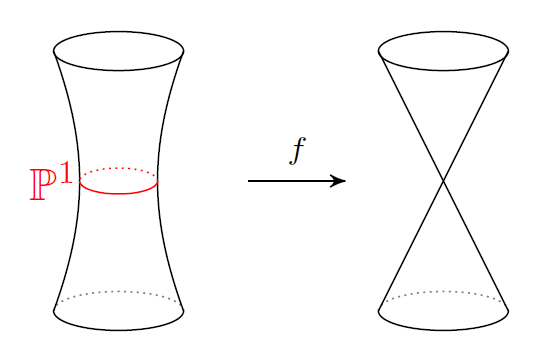Resolutions of singularities
Noncommutative symplectic geometry, formally smooth algebras, derived equivalences between noncommutative algebras and algebraic varieties.
•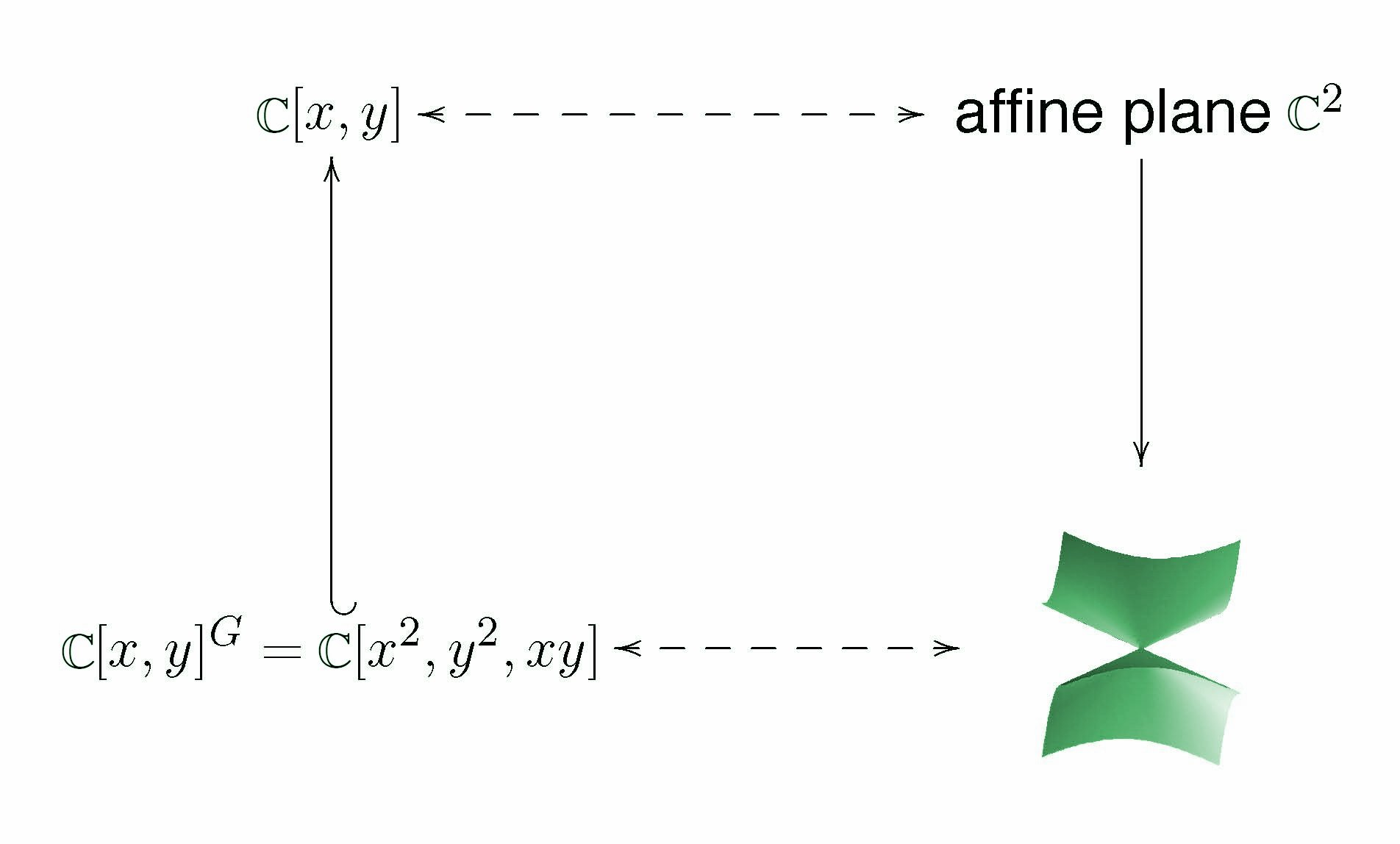Geometric invariant theory
quotient singularities, moduli spaces of stable representations, slice theorems. Stability conditions.
•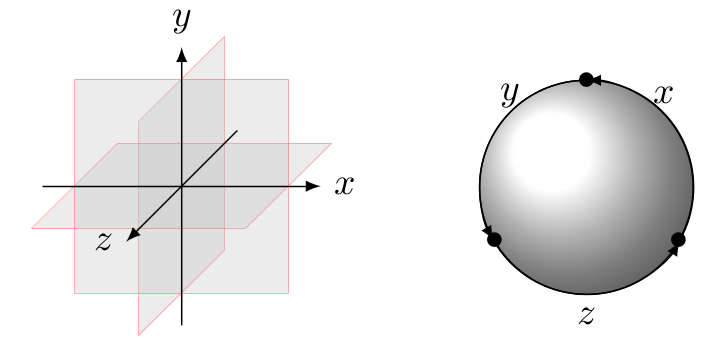Homological mirror symmetry
Matrix factorizations for noncommutative algebras, Fukaya categories of (punctured) surfaces.
•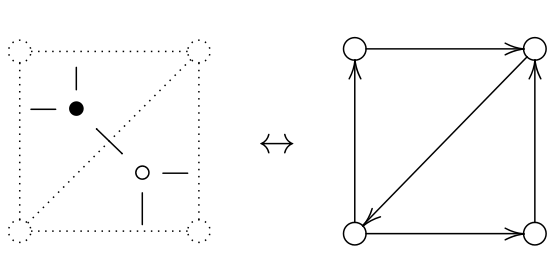Dimer models
Representations of Jacobi Algebras, Noncomutative crepant resolutions and the relation to mirror symmetry

## Books

•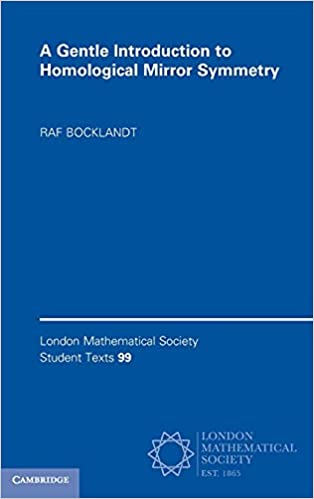A Gentle Introduction to Homological Mirror Symmetry
I have written an introductory book on Homological Mirror symmetry aimed at graduate students. The book is written from the perspective of representation theory and illustrates the important concepts in Mirror symmetry using examples coming from quivers and Fukaya categories of surfaces. The book is published by Cambridge university press. If you are interested you can read its table of content and a sample excerpt.

•A Journey through Spaces and Time
I am working on a book project which tells the story of geometry from Euclid to Grothendieck by visiting the historical places where geometry was important, such as Ancient Alexandria, Medieval Florence, Revolutionary Paris, Fascist Rome, etc. If you're interested, you can read two excerpts here.

## Contact

• University of Amsterdam
Korteweg-de Vries Institute for Mathematics
Science Park 105-107
1098 XG Amsterdam
The Netherlands
Tel: +31 (0) 20 525 6553
Room: F3.13
Email: raf.bocklandt"at"gmail.com